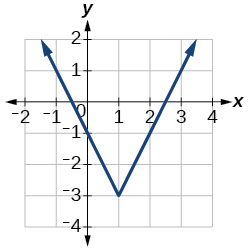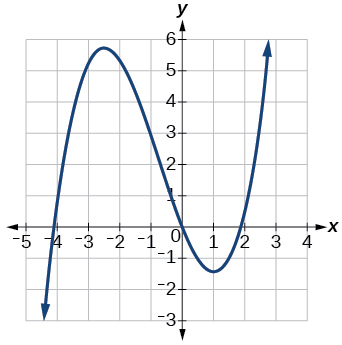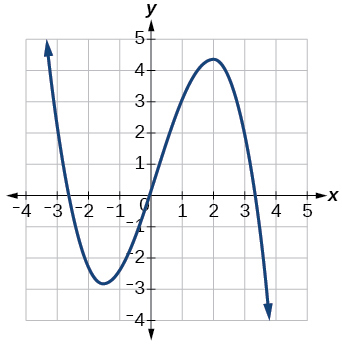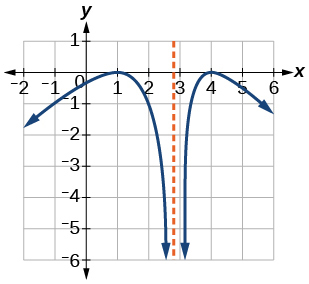3.3 Rates of change and behavior of graphs  (Page 5/15)

 Page 5 / 15

Access this online resource for additional instruction and practice with rates of change.

Key equations

 Average rate of change $\frac{\Delta y}{\Delta x}=\frac{f\left({x}_{2}\right)-f\left({x}_{1}\right)}{{x}_{2}-{x}_{1}}$

Key concepts

• A rate of change relates a change in an output quantity to a change in an input quantity. The average rate of change is determined using only the beginning and ending data. See [link] .
• Identifying points that mark the interval on a graph can be used to find the average rate of change. See [link] .
• Comparing pairs of input and output values in a table can also be used to find the average rate of change. See [link] .
• An average rate of change can also be computed by determining the function values at the endpoints of an interval described by a formula. See [link] and [link] .
• The average rate of change can sometimes be determined as an expression. See [link] .
• A function is increasing where its rate of change is positive and decreasing where its rate of change is negative. See [link] .
• A local maximum is where a function changes from increasing to decreasing and has an output value larger (more positive or less negative) than output values at neighboring input values.
• A local minimum is where the function changes from decreasing to increasing (as the input increases) and has an output value smaller (more negative or less positive) than output values at neighboring input values.
• Minima and maxima are also called extrema.
• We can find local extrema from a graph. See [link] and [link] .
• The highest and lowest points on a graph indicate the maxima and minima. See [link] .

Verbal

Can the average rate of change of a function be constant?

Yes, the average rate of change of all linear functions is constant.

If a function $\text{\hspace{0.17em}}f\text{\hspace{0.17em}}$ is increasing on $\text{\hspace{0.17em}}\left(a,b\right)\text{\hspace{0.17em}}$ and decreasing on $\text{\hspace{0.17em}}\left(b,c\right),\text{\hspace{0.17em}}$ then what can be said about the local extremum of $\text{\hspace{0.17em}}f\text{\hspace{0.17em}}$ on $\text{\hspace{0.17em}}\left(a,c\right)?\text{\hspace{0.17em}}$

How are the absolute maximum and minimum similar to and different from the local extrema?

The absolute maximum and minimum relate to the entire graph, whereas the local extrema relate only to a specific region around an open interval.

How does the graph of the absolute value function compare to the graph of the quadratic function, $\text{\hspace{0.17em}}y={x}^{2},\text{\hspace{0.17em}}$ in terms of increasing and decreasing intervals?

Algebraic

For the following exercises, find the average rate of change of each function on the interval specified for real numbers $\text{\hspace{0.17em}}b\text{\hspace{0.17em}}$ or $\text{\hspace{0.17em}}h$ in simplest form.

$f\left(x\right)=4{x}^{2}-7\text{\hspace{0.17em}}$ on

$4\left(b+1\right)$

$g\left(x\right)=2{x}^{2}-9\text{\hspace{0.17em}}$ on

$p\left(x\right)=3x+4\text{\hspace{0.17em}}$ on

3

$k\left(x\right)=4x-2\text{\hspace{0.17em}}$ on

$f\left(x\right)=2{x}^{2}+1\text{\hspace{0.17em}}$ on $\text{\hspace{0.17em}}\left[x,x+h\right]$

$4x+2h$

$g\left(x\right)=3{x}^{2}-2\text{\hspace{0.17em}}$ on $\text{\hspace{0.17em}}\left[x,x+h\right]$

$a\left(t\right)=\frac{1}{t+4}\text{\hspace{0.17em}}$ on $\text{\hspace{0.17em}}\left[9,9+h\right]$

$\frac{-1}{13\left(13+h\right)}$

$b\left(x\right)=\frac{1}{x+3}\text{\hspace{0.17em}}$ on $\text{\hspace{0.17em}}\left[1,1+h\right]$

$j\left(x\right)=3{x}^{3}\text{\hspace{0.17em}}$ on $\text{\hspace{0.17em}}\left[1,1+h\right]$

$3{h}^{2}+9h+9$

$r\left(t\right)=4{t}^{3}\text{\hspace{0.17em}}$ on $\text{\hspace{0.17em}}\left[2,2+h\right]$

$\frac{f\left(x+h\right)-f\left(x\right)}{h}\text{\hspace{0.17em}}$ given $\text{\hspace{0.17em}}f\left(x\right)=2{x}^{2}-3x\text{\hspace{0.17em}}$ on $\text{\hspace{0.17em}}\left[x,x+h\right]$

$4x+2h-3$

Graphical

For the following exercises, consider the graph of $\text{\hspace{0.17em}}f\text{\hspace{0.17em}}$ shown in [link] .

Estimate the average rate of change from $\text{\hspace{0.17em}}x=1\text{\hspace{0.17em}}$ to $\text{\hspace{0.17em}}x=4.$

Estimate the average rate of change from $\text{\hspace{0.17em}}x=2\text{\hspace{0.17em}}$ to $\text{\hspace{0.17em}}x=5.$

$\frac{4}{3}$

For the following exercises, use the graph of each function to estimate the intervals on which the function is increasing or decreasing.increasing on $\text{\hspace{0.17em}}\left(-\infty ,-2.5\right)\cup \left(1,\infty \right),\text{\hspace{0.17em}}$ decreasing onincreasing on $\text{\hspace{0.17em}}\left(-\infty ,1\right)\cup \left(3,4\right),\text{\hspace{0.17em}}$ decreasing on $\text{\hspace{0.17em}}\left(1,3\right)\cup \left(4,\infty \right)$

For the following exercises, consider the graph shown in [link] .

sin^4+sin^2=1, prove that tan^2-tan^4+1=0
what is the formula used for this question? "Jamal wants to save \$54,000 for a down payment on a home. How much will he need to invest in an account with 8.2% APR, compounding daily, in order to reach his goal in 5 years?"
i don't need help solving it I just need a memory jogger please.
Kuz
A = P(1 + r/n) ^rt
Dale
how to solve an expression when equal to zero
its a very simple
Kavita
gave your expression then i solve
Kavita
Hy guys, I have a problem when it comes on solving equations and expressions, can you help me 😭😭
Thuli
Tomorrow its an revision on factorising and Simplifying...
Thuli
ok sent the quiz
kurash
send
Kavita
Hi
Masum
What is the value of log-1
Masum
the value of log1=0
Kavita
Log(-1)
Masum
What is the value of i^i
Masum
log -1 is 1.36
kurash
No
Masum
no I m right
Kavita
No sister.
Masum
no I m right
Kavita
tan20°×tan30°×tan45°×tan50°×tan60°×tan70°
jaldi batao
Joju
Find the value of x between 0degree and 360 degree which satisfy the equation 3sinx =tanx
what is sine?
what is the standard form of 1
1×10^0
Akugry
Evalute exponential functions
30
Shani
The sides of a triangle are three consecutive natural number numbers and it's largest angle is twice the smallest one. determine the sides of a triangle
Will be with you shortly
Inkoom
3, 4, 5 principle from geo? sounds like a 90 and 2 45's to me that my answer
Neese
answer is 2, 3, 4
Gaurav
prove that [a+b, b+c, c+a]= 2[a b c]
can't prove
Akugry
i can prove [a+b+b+c+c+a]=2[a+b+c]
this is simple
Akugry
hi
Stormzy
x exposant 4 + 4 x exposant 3 + 8 exposant 2 + 4 x + 1 = 0
x exposent4+4x exposent3+8x exposent2+4x+1=0
HERVE
How can I solve for a domain and a codomains in a given function?
ranges
EDWIN
Thank you I mean range sir.
Oliver
proof for set theory
don't you know?
Inkoom
find to nearest one decimal place of centimeter the length of an arc of circle of radius length 12.5cm and subtending of centeral angle 1.6rad
factoring polynomialByByBy